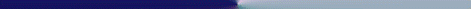## Using Formulas Tutorial

Use SUMIF instead of D... functions
With SUMIF, you don't need ot set up a criteria range. If your sum criteria is simple, you can include it in the SUMIF statement.

Comparative Ratio Analysis
You can use Excel formulas to compare actual data to Robert Morris Associates annual statement studies. RMA comparative ratios are available in the reference section of most business libraries. See the sheet named RMA for more information. Lending institutions use the RMA ratios as one measure of a business that is applying for a loan. By becoming familiar with the RMA ratiios, you can improve the financial measures of your business - before it is time to apply for a loan.

Indicator Lights
Without any VBA code, you can create an indicator light that turns red or green depending on the value of an Excel variable. The secret is to create two cells on a hidden sheet - one green and one red - each containing the value of a third cell. Then create a linked picture of one of the cells. So, now you have an indicator light of one color...until you create a named formula (read about these in on-line help). Set up the named formula so that it returns the value of either the red or green cell - something like this:

=IF(SomeCellValue>LimitValue,RedCell,GreenCell)

Finally change the link for the picture to equal the named formula.

When a cell values change, the value of the named formula changes...the picture is linked to the value of the named formula and the linked picture changes - red if the cell value is greater than the limit, green if not.

Create a Year To Date Total
Let's say you have a worksheet with 12 columns of monthly data...and you want to create a new column with a year to date total that will update automatically every time you change the "current month date" at the top of the worksheet.

Name the current month date "CURRENTMONTH" and use a formula like this to create a YTD total:
=SUM(B4:OFFSET(B4,,MONTH(CURRENTMONTH)-1))
where the first column of monthly data is in column B.

To return the current month's value to a column, use this formula:
=OFFSET(B4,,MONTH(CURRENTMONTH)-1)

Each time you change the date in the range "CURRENTMONTH", the offset function will cause Excel to find the current month's data.

Use F9 to Look Inside a Formula
When reviewing a formula on the formula bar, you can "look inside" the calculation by selecting a portion of the formula and pressing F9. Excel will display the value of that portion of the formula. This technique is particularly helpful for understanding and debugging array formulas. Careful, though...after viewing the value, press ESC - otherwise, Excel will replace part of your formula with the value.

Take a shortcut with SUMIF
When you need to analyze a list of data then you can set up criteria ranges and use database functions like DSUM, DAVERAGE or DCOUNT

- OR, take a shortcut and use SUMIF and COUNTIF.  These functions have built in criteria ranges, so you save time.

=SUMIF(C16:C:45,">10.2",G16:G45) will sum all the values in column G where column C is greater than 10.2.

=COUNTIF(C16:C45,">10.2",G16:G45) will count the number of rows where C is greater than 10.2